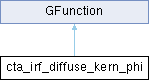GammaLib  1.7.0.dev
cta_irf_diffuse_kern_phi Class Reference

Kernel for IRF azimuth angle integration of the diffuse source model. More...

#include <GCTAResponse_helpers.hpp>

Inheritance diagram for cta_irf_diffuse_kern_phi:## Public Member Functions

cta_irf_diffuse_kern_phi (const GCTAResponseIrf *rsp, const GModelSpatial *model, const GMatrix *rot, const double &zenith, const double &azimuth, const GEnergy &srcEng, const GTime &srcTime, const double &srcLogEng, const GEnergy &obsEng, const double &sin_theta, const double &cos_theta, const double &sin_ph, const double &cos_ph)

double eval (const double &phi)
Kernel for IRF azimuth angle integration of the diffuse source model. More...Public Member Functions inherited from GFunction
GFunction (void)
Void constructor. More...

GFunction (const GFunction &function)
Copy constructor. More...

virtual ~GFunction (void)
Destructor. More...

GFunctionoperator= (const GFunction &function)
Assignment operator. More...

## Protected Attributes

const GCTAResponseIrfm_rsp
CTA response. More...

const GModelSpatialm_model
Spatial model. More...

const GMatrixm_rot
Rotation matrix. More...

double m_zenith
Zenith angle. More...

double m_azimuth
Azimuth angle. More...

GEnergy m_srcEng
True photon energy. More...

GTime m_srcTime
True photon arrival time. More...

double m_srcLogEng
True photon log energy. More...

GEnergy m_obsEng
Measured event energy. More...

double m_sin_theta
Sine of offset angle. More...

double m_cos_theta
Cosine of offset angle. More...

double m_sin_ph
Sine term in angular distance equation. More...

double m_cos_ph
Cosine term in angular distance equation. More...

GVector m_native
Pre-allocate memory for native coordinates. More...

GPhoton m_photon
Pre-allocate memory for a photon object. More...Protected Member Functions inherited from GFunction
void init_members (void)
Initialise class members. More...

void copy_members (const GFunction &function)
Copy class members. More...

void free_members (void)
Delete class members. More...

## Detailed Description

Kernel for IRF azimuth angle integration of the diffuse source model.

This class implements the computation of

$S_{\rm p}(\theta, \phi | E, t) \, Aeff(\theta, \phi) \, Edisp(\theta, \phi)$

where

• $$S_{\rm p}(\theta, \phi | E, t)$$ is the diffuse model,
• $$Aeff(\theta, \phi)$$ is the effective area,
• $$Edisp(\theta, \phi)$$ is the energy dispersion,
• $$\theta$$ is the distance from the PSF centre, and
• $$\phi$$ is the azimuth angle.

Definition at line 849 of file GCTAResponse_helpers.hpp.

## Constructor & Destructor Documentation

 cta_irf_diffuse_kern_phi::cta_irf_diffuse_kern_phi ( const GCTAResponseIrf * rsp, const GModelSpatial * model, const GMatrix * rot, const double & zenith, const double & azimuth, const GEnergy & srcEng, const GTime & srcTime, const double & srcLogEng, const GEnergy & obsEng, const double & sin_theta, const double & cos_theta, const double & sin_ph, const double & cos_ph )
inline

Definition at line 851 of file GCTAResponse_helpers.hpp.

## Member Function Documentation

 double cta_irf_diffuse_kern_phi::eval ( const double & phi )
virtual

Kernel for IRF azimuth angle integration of the diffuse source model.

Parameters
 [in] phi Azimuth angle around observed photon direction (radians).

Computes

$S_{\rm p}(\theta, \phi | E, t) \, Aeff(\theta, \phi) \, Edisp(\theta, \phi)$

As the coordinates $$(\theta, \phi)$$ are given in the reference frame of the observed photon direction, some coordinate transformations have to be performed.

First, $$(\theta, \phi)$$ are transformed into the celestial reference frame using the rotation matrix.

Then, the offset angle of the true photon direction is computed in the camera system (so far we do not compute the azimuth angle as we assume an azimuthally symmetric response).

Todo:

Optimize computation of sky direction in native coordinates

Implement azimuth angle computation of true photon in camera

Replace (theta,phi) by (delta,alpha)

Implements GFunction.

Definition at line 1400 of file GCTAResponse_helpers.cpp.

## Member Data Documentation

 double cta_irf_diffuse_kern_phi::m_azimuth
protected

Azimuth angle.

Definition at line 885 of file GCTAResponse_helpers.hpp.

Referenced by eval().

 double cta_irf_diffuse_kern_phi::m_cos_ph
protected

Cosine term in angular distance equation.

Definition at line 893 of file GCTAResponse_helpers.hpp.

Referenced by eval().

 double cta_irf_diffuse_kern_phi::m_cos_theta
protected

Cosine of offset angle.

Definition at line 891 of file GCTAResponse_helpers.hpp.

Referenced by eval().

 const GModelSpatial* cta_irf_diffuse_kern_phi::m_model
protected

Spatial model.

Definition at line 882 of file GCTAResponse_helpers.hpp.

Referenced by eval().

 GVector cta_irf_diffuse_kern_phi::m_native
protected

Pre-allocate memory for native coordinates.

Definition at line 894 of file GCTAResponse_helpers.hpp.

Referenced by eval().

 GEnergy cta_irf_diffuse_kern_phi::m_obsEng
protected

Measured event energy.

Definition at line 889 of file GCTAResponse_helpers.hpp.

Referenced by eval().

 GPhoton cta_irf_diffuse_kern_phi::m_photon
protected

Pre-allocate memory for a photon object.

Definition at line 895 of file GCTAResponse_helpers.hpp.

Referenced by eval().

 const GMatrix* cta_irf_diffuse_kern_phi::m_rot
protected

Rotation matrix.

Definition at line 883 of file GCTAResponse_helpers.hpp.

Referenced by eval().

 const GCTAResponseIrf* cta_irf_diffuse_kern_phi::m_rsp
protected

CTA response.

Definition at line 881 of file GCTAResponse_helpers.hpp.

Referenced by eval().

 double cta_irf_diffuse_kern_phi::m_sin_ph
protected

Sine term in angular distance equation.

Definition at line 892 of file GCTAResponse_helpers.hpp.

Referenced by eval().

 double cta_irf_diffuse_kern_phi::m_sin_theta
protected

Sine of offset angle.

Definition at line 890 of file GCTAResponse_helpers.hpp.

Referenced by eval().

 GEnergy cta_irf_diffuse_kern_phi::m_srcEng
protected

True photon energy.

Definition at line 886 of file GCTAResponse_helpers.hpp.

Referenced by eval().

 double cta_irf_diffuse_kern_phi::m_srcLogEng
protected

True photon log energy.

Definition at line 888 of file GCTAResponse_helpers.hpp.

Referenced by eval().

 GTime cta_irf_diffuse_kern_phi::m_srcTime
protected

True photon arrival time.

Definition at line 887 of file GCTAResponse_helpers.hpp.

Referenced by eval().

 double cta_irf_diffuse_kern_phi::m_zenith
protected

Zenith angle.

Definition at line 884 of file GCTAResponse_helpers.hpp.

Referenced by eval().

The documentation for this class was generated from the following files: